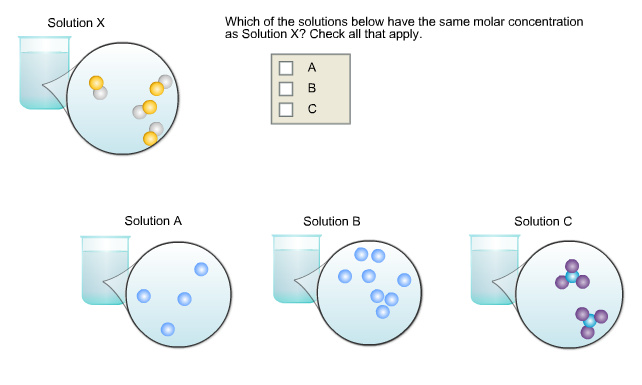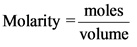# Which of the solutions below have the same molar concentration as Solution X? Check all that apply

Which of the solutions below have the same molar concentration as Solution X? Check all that apply.Concepts and reason
Molarity is the number of moles of solute in one liter of the solution. Mathematically, it can be expressed as follows:Fundamentals
Concentration of a solution can be expressed in terms likes molarity, normality, molality, and mole fraction. Molarity and normality are the widely used terms to express the concentration of a solution. Avogadro number of particles is called as one mole.

Consider the following solutions:

Number of species in unit volume in solution X and solution A are equal.

Explanation:
In solution X, there are four species of solute in unit volume. In solution A, there are 4 species of solute, solution B has 8 species of solute and solution C has 2 species of solute.

If the number of moles present in unit volume increases, its concentration increases.
The number of moles of solution A is equal to the number of moles of solution X. Hence, the solution A has same molar concentration as solution X.Explanation:
Solution B has more number of species than solution X. Hence, the molar concentration of solution B is higher than the solution X. Solution C has less number of moles in unit than solution C. Hence, the molar concentration of solution C is lower than the molar concentration of solution X.